# SSAT Upper Level Math : Number Concepts and Operations

## Example Questions

1 2 49 50 51 52 53 54 55 57 Next →

### Example Question #561 : Number Concepts And Operations

In a small school, ninth grade comprises 50 students, including 18 boys. Each student is assigned to one of two home rooms - those of Mr. Jones and Mrs. Wilson. The boys and the girls are both divided evenly between the home rooms.

According to the charter, two of the student council representatives from the ninth grade will be selected by choosing one at random from each home room. What are the odds of one boy and one girl being selected?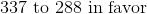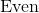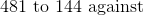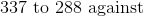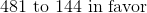Explanation:

18 out of the 50 students are boys, so 32 are girls. Also, since the boys and the girls are split evenly between the home rooms, each home room has 9 boys and 16 girls for a total of 25 students.

This means that the probability of a randomly selected student from Mr. Jones's home room being a boy is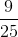, and that of the student being a girl is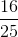. These probabilities are the same for Mrs. Wilson's class.

Therefore, the probability of one boy being selected from Mr. Jones's class and one girl being selected from Mrs. Wilson's class is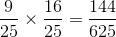; the probability of the reverse event is the same. Therefore, the probability of one boy and one girl being selected is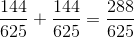The odds against this happening are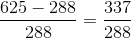- that is, 337 to 288.

### Example Question #561 : Number Concepts And Operations

If Bob rolled 3 fair six sided dice, what is the probability of NOT rolling a sum of 3?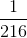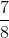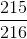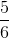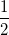Explanation:

The only possible combination to roll a sum of 3 is by rolling three 1s.  The probability of rolling any side of a die is.

The odds of rolling three 1s are: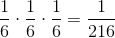Therefore, the probability of NOT rolling three 1s is: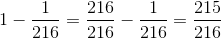1 2 49 50 51 52 53 54 55 57 Next →

### All SSAT Upper Level Math Resources